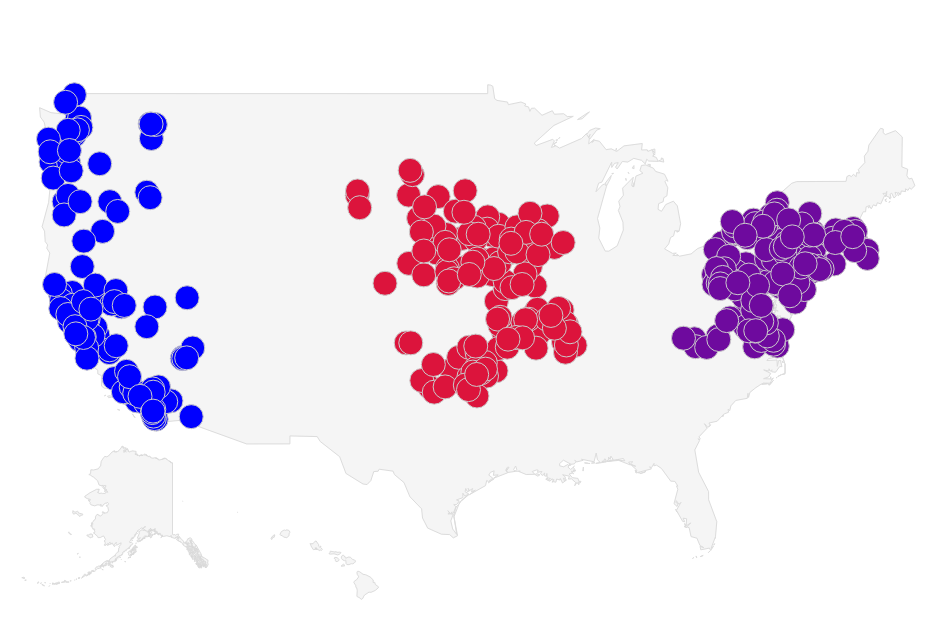# K-means Clustering With Ruby

Let’s say we have a collection of businesses in three distinct regions: the East coast, West coast and fly-over country, and we would like to classify these businesses by region. Clustering algorithms, which aim to partition a set of data points into a small number of clusters, are some of the most commonly used solutions for data classification problems.

The k-means algorithm is what we will use to cluster our data. It is an unsupervised machine learning algorithm that groups data points into k clusters, minimizing the distance from each data point to the centroid of the cluster it belongs to.

### The Algorithm

1. Choose k points to represent the initial centroids for the data. These may be assigned randomly or specified by the user.
2. Assign each data point to the closest centroid (by euclidean distance or other distance metric), creating k clusters.
3. Recalculate each centroid’s position such that each data point in the centroid’s cluster is equidistant to the centroid.
4. Repeat steps 2 and 3 until convergence (no data points change cluster) or up to a maximum number of iterations.

### The Code

Let’s start by implementing classes to store the businesses, centroids and clusters. The `DataPoint` class will represent the businesses and centroids. A `DataPoint` should be able to do the following:

• Store the latitude, longitude and state belonging to a business.
• Calculate the distance between itself and another point or centroid.

Since we are dealing with geographical coordinates, for this implementation I’ve elected to use the geographic distance between points instead of the euclidean distance, using utilities from the Geocoder library. I would like to pass instances of the `DataPoint` class to the Geocoder library for distance calculations, so we will also need to implement an instance method, `to_coordinates`, that returns an array of latitude and longitude.

To store the data points, we will use a `Set` because it will make cluster comparison easier (for step 4 of the algorithm). The Ruby `Set` class uses the `eql?` and `hash` methods for equality comparisons, so our `DataPoint` class will also need to implement them.

Now that we have the basic gist of the requirements for the `DataPoint` class, let’s write a few test cases to assert them.

The class implementation is pretty simple.

Up next is the `Cluster` class, which we will use to perform the following functions:

• Store a centroid and a set of data points.
• Recalculate the position of the centroid to make it equidistant to all points in the cluster within a very small margin of error.
• Implement a method to compare itself with another cluster.

With these specifications in mind, we write the tests:

Followed by the class implementation:

Finally, we implement the algorithm in a class called `Clusterer`. This class needs to run through the four steps of the algorithm and terminate on convergence or after a maximum number of iterations. It should also export the clustered data in a format that we can plot on a map. Let’s assert these requirements.

Here’s an abridged version of the algorithm’s implementation, showcasing the meat of the algorithm, which is pretty straightforward. The full `Clusterer` class is here.

### Results

Since the k-means algorithm does not guarantee to find the global optimum, we can’t expect that the data will be clustered in the same way after each run. However, given that we have some knowledge of the data we are clustering, we can provide initial centroids that will result in the global optimum. For the chart below I picked 500 businesses in groupings of states to make the clustering easier. I also chose the initial centroids to ensure that the algorithm found the global optimum. I plotted the chart using a Google Visualization.You can find the complete code for this blog post in my algorithms repository on github.